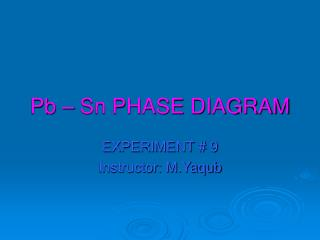DownloadDownload PresentationPb – Sn PHASE DIAGRAM

# Pb – Sn PHASE DIAGRAM

Download Presentation## Pb – Sn PHASE DIAGRAM

- - - - - - - - - - - - - - - - - - - - - - - - - - - E N D - - - - - - - - - - - - - - - - - - - - - - - - - - -
##### Presentation Transcript

1. Pb – Sn PHASE DIAGRAM EXPERIMENT # 9 Instructor: M.Yaqub

2. SOLUTION • Solid solution: Consists of atoms ofat least twodifferent types • Solute an element or compound present in a minor concentration • Solvent  an element or compound in greater amount; host atoms. • Solubility Limit: The maximum concentration of a solute atoms that may dissolve in the solvent to form a solid solution at some specific temperature.

3. PHASE • Phase:defined as a homogeneous portion of a system that has uniform physical and chemical characteristics. • Everypure materialis considered to be a phase • Also every solid, liquid, and gaseous solution • e.g., syrup solution is one phase, and solid sugar is another

4. PHASE DIAGRAM • Equilibrium Phase diagram: • Represents the relationships between temperature and the compositions and the quantities of phases at equilibrium. • Also known as phase, equilibrium or constitutional diagram • Abinary alloyis one that contains two components.

5. Pb – Sn PHASE DIAGRAM • a - Solid solution of tin in lead (tin “Sn” is solute and lead “Pb” is solvent) • Solubility limit is 18.3% at 183 oC • b - Solid solution of lead in tin (lead “Pb” is solute and tin “Sn” is solvent) • Solubility limit is 2.2% at 183 oC • Eutectic point at 61.9% Sn at 183 oC

6. Pb – Sn PHASE DIAGRAM

7. DIFFERENTIAL SCANNING CALORIMETR • Differential Scanning Calorimeter (DSC) is used to make a phase diagram. • Read the hand out for detail procedure.

8. PLOT OF HEAT FLOW

9. LAB REPORT • From the resultant DSC curves determine the following parameters: • Initial melting temperature for each sample. • Melting peak for each sample. • Final melting point for each sample. • Calculate the heat of fusion, which is the area under the curve between the solid and the dotted lines for each sample.

10. LAB REPORT (Continue) • Plot the measured transition temperatures on the Pb-Sn phase diagram. • Tabulate all values for all tested specimens in a table format. • Estimate the mass fractions of each phase in the microstructure given below. • Predict the equilibrium mass fractions of each phase using the phase diagram and the lever rule.